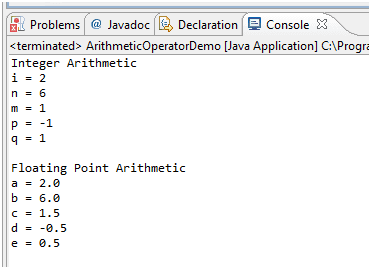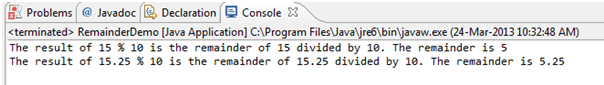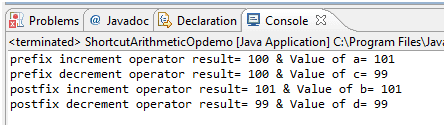# Java Arithmetic Operators

## Description

We can use arithmetic operators to perform calculations with values in programs. Arithmetic operators are used in mathematical expressions in the same way that they are used in algebra. A value used on either side of an operator is called an operand. For example, in below statement the expression 47 + 3, the numbers 47 and 3 are operands. The arithmetic operators are examples of binary operators because they require two operands. The operands of the arithmetic operators must be of a numeric type. You cannot use them on boolean types, but you can use them on char types, since the char type in Java is, essentially, a subset of int.

```int a = 47+3;
```
Operator Use Description Example
+ x + y Adds x and y float num = 23.4 + 1.6; // num=25
- x - y Subtracts y from x long n = 12.456 – 2.456; //n=10
-x Arithmetically negates x int i = 10; -i; // i = -10
* x * y Multiplies x by y int m = 10*2; // m=20
/ x / y Divides x by y float div = 20/100 ; // div = 0.2
% x % y Computes the remainder of dividing x by y int rm = 20/3; // rm = 2

In Java, you need to be aware of the type of the result of a binary (two-argument) arithmetic operator.

• If either operand is of type double, the other is converted to double.
• Otherwise, if either operand is of type float, the other is converted to float.
• Otherwise, if either operand is of type long, the other is converted to long.
• Otherwise, both operands are converted to type int.

For unary (single-argument) arithmetic operators:

• If the operand is of type byte, short, or char then the result is a value of type int.
• Otherwise, a unary numeric operand remains as is and is not converted.

The basic arithmetic operations—addition, subtraction, multiplication, and division— all behave as you would expect for all numeric types. The minus operator also has a unary form that negates its single operand. Remember that when the division operator is applied to an integer type, there will be no fractional component attached to the result.

The following simple program demonstrates the arithmetic operators. It also illustrates the difference between floating-point division and integer division.

Java Code: Go to the editor

``````public class ArithmeticOperatorDemo {
// Demonstrate the basic arithmetic operators.
public static void main(String args[]) {
// arithmetic using integers
System.out.println("Integer Arithmetic");
int i = 1 + 1;
int n = i * 3;
int m = n / 4;
int p = m - i;
int q = -p;
System.out.println("i = " + i);
System.out.println("n = " + n);
System.out.println("m = " + m);
System.out.println("p = " + p);
System.out.println("q = " + q);
// arithmetic using doubles
System.out.println("\nFloating Point Arithmetic");
double a = 1 + 1;
double b = a * 3;
double c = b / 4;
double d = c - a;
double e = -d;
System.out.println("a = " + a);
System.out.println("b = " + b);
System.out.println("c = " + c);
System.out.println("d = " + d);
System.out.println("e = " + e);
}
}
```
```

Output:The Modulus Operator

The modulus operator, %, returns the remainder of a division operation. It can be applied to floating-point types as well as integer types. The following example program demonstrates the %:

Java Code: Go to the editor

``````public class RemainderDemo {
public static void main (String [] args) {
int x = 15;
int int_remainder = x % 10;
System.out.println("The result of 15 % 10 is the "
+ "remainder of 15 divided by 10. The remainder is " + int_remainder);
double d = 15.25;
double double_remainder= d % 10;
System.out.println("The result of 15.25 % 10 is the "
+ "remainder of 15.25 divided by 10. The remainder is " + double_remainder);
}
}
```
```

Output:Also, there are a couple of quirks to keep in mind regarding division by 0:

• A non-zero floating-point value divided by 0 results in a signed Infinity. 0.0/0.0 results isNaN.
• A non-zero integer value divided by integer 0 will result in ArithmeticException at runtime

Shortcut Arithmetic Operators (Increment and decrement operator)

The increment operator increases its operand by one. The decrement operator decreases its operand by one. For example, this statement:

`x = x + 1;`
`x++;`

Same way decrement operator

`x = x - 1;`

is equivalent to

`x--;`

These operators are unique in that they can appear both in postfix form, where they follow the operand as just shown, and prefix form, where they precede the operand. In the foregoing examples, there is no difference between the prefix and postfix forms. However, when the increment and/or decrement operators are part of a larger expression, then a subtle, yet powerful, difference between these two forms appears. In the prefix form, the operand is incremented or decremented before the value is obtained for use in the expression. In postfix form, the previous value is obtained for use in the expression, and then the operand is modified.Let’s understand this concept with help of example below,

Java Code: Go to the editor

```public class ShortcutArithmeticOpdemo {
public static void main(String[] args) {
int a,b,c,d;
a=b=c=d=100;

int p = a++;
int r = c--;
int q = ++b;
int s = --d;
System.out.println("prefix increment operator result= "+ p + " & Value of a= "+ a);
System.out.println("prefix decrement operator result= "+ r + " & Value of c= "+c);
System.out.println("postfix increment operator result= "+ q + " & Value of b= "+ b);
System.out.println("postfix decrement operator result= "+ s + " & Value of d= "+d);
}
}
```

Output:Summary

• Arithmetic operators are used in mathematical expressions.
• Arithmetic operators are +(addition) , -(subtraction), * (multiplication), / (division) and % (reminder).
• Java provides built-in short-circuit addition and subtraction operators.

Java Code Editor:

Previous: Assignment Operator
Next: Conditional Operator

﻿